## How to use vlookup exact and approximate match in Excel?

In Excel, vlookup is one of the most important functions for us to search a value in the left-most column of the table and return the value in the same row of the range. But, do you apply the vlookup function successfully in Excel? This article, I will talk about how to use the vlookup function in Excel.

Use vlookup function to get the exact matches in Excel

Use vlookup function to get the approximate matches in Excel

Vlookup to get the exact matches with a handy feature

#### Use vlookup function to get the exact matches in Excel

First, you must know the vlookup syntax and details of the parameters.

The syntax of VLOOKUP function in Excel is:

VLOOKUP(lookup_value, table_array, col_index_num, [range_lookup])
• lookup_value: the value to search based on in the first column of the table;
• table_array: the range of cells that contains the data, you can use a reference to a range such as A1:D10, or a range name;
• col_index_num: the column number in the table_array argument from which the matching value must be returned;
• range_lookup: is optional operation, FALSE to find an exact match, and TRUE to find the approximate match.

Now, I will take an example to explain this vlookup function to get the exact matches, supposing you have the following list of persons’ information in range A2:D12: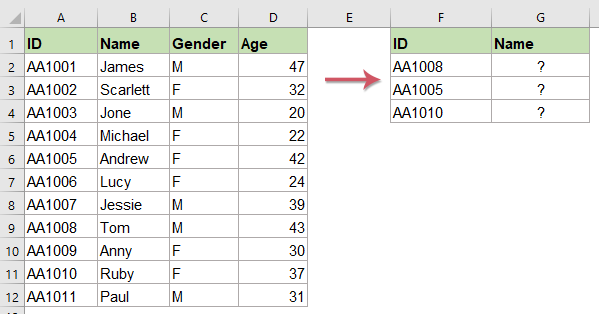In this case, you want to find the corresponding names with the IDs in the same row, please use the following vlookup formula into a blank cell where you want to get the result:

=VLOOKUP(F2,\$A\$2:\$D\$12,2,FALSE)

Then, drag the fill handle down to the cells to apply this formula, and you will return the results as below screenshot shown: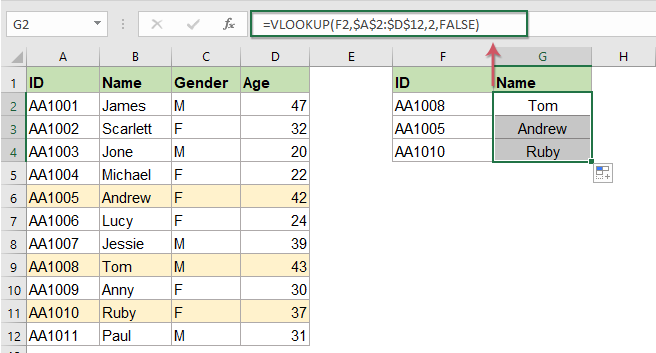Notes:

1. In the above formula: F2 is the value which you want to return its relative information, A2:D12 is the data range you use, the number 2 indicates the column number that your matched value is returned and the FALSE refers to the exact match.

2. If your criteria value is not found in the data range, you will get an error value #N/A.

If you are interested in VLOOKUP function in Excel, Kutools for Excel's Super LOOKUP supports some powerful Vlookup formulas for you, you can quickly do the Vlookup function without remembering any formulas. Click to download Kutools for Excel!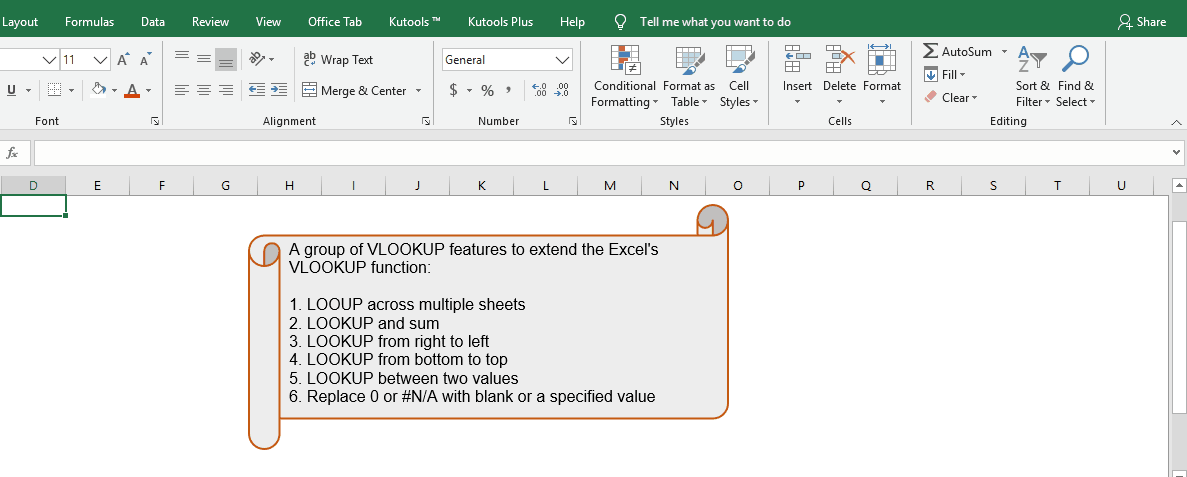Kutools for Excel: with more than 300 handy Excel add-ins, free to try with no limitation in 30 days. Download and free trial Now!

#### Use vlookup function to get the approximate matches in Excel

Sometimes, your specified data is not in the data range, to get the nearest match with the given data, you need to use the vlookup to get an approximate match.

If you have the following range data, the specified quantity number 58 is not in the Quantity column, how to get its closest unit price in column B?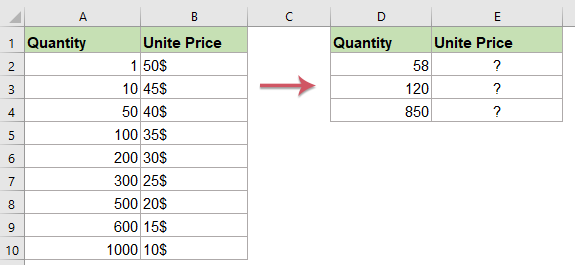Please enter the below formula into a blank cell:

=VLOOKUP(D2,\$A\$2:\$B\$10,2,TRUE)

Then, drag the fill handle down to the cells to apply this formula, and you will get the approximate matches based on the given values, see screenshot: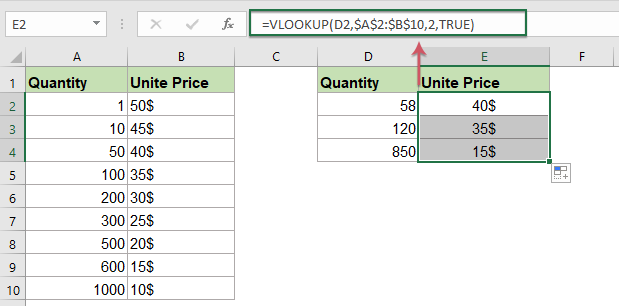Notes:

1. In the above formula: D2 is the value which you want to return its relative information, A2:B10 is the data range you use, the number 2 indicates the column number that your matched value is returned and the TRUE refers to the approximate match.

2. The approximate match returns the next largest value that is less than your specific lookup value.

3. To use the vlookup function to get an approximate match value, your first column in the table must be sorted in ascending order, otherwise it will return a wrong result.

#### Vlookup to get the exact matches with a handy feature

If you have Kutools for Excel, with its Look for a value in list formula, you can quickly return the matching data based on the specific values.

Note: To apply this Look for a value in list, firstly, you should download the Kutools for Excel, and then apply the feature quickly and easily.

After installing Kutools for Excel, please do as this:

1. Click a cell where you want to put the matched result.

2. Then click Kutools > Formula Helper > Formula Helper, see screenshot: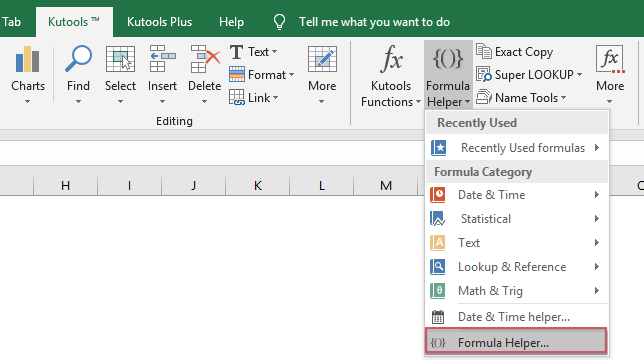3. In the Formulas Helper dialog box, please do the following operations:

(1.) In the Formula Type drop down list, please select Lookup option;

(2.) Then, select Look for a value in list option in the Choose a formula list box;

(3.)  And then, in the Arguments input text boxes, please do the following operations:

• Click first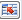button beside Table_array to select the data range which you want to use;
• Click secondbutton beside Lookup_value to select the criteria that you want to return its matched value;
• Click thirdbutton beside Column to select the column contains the value you want to return.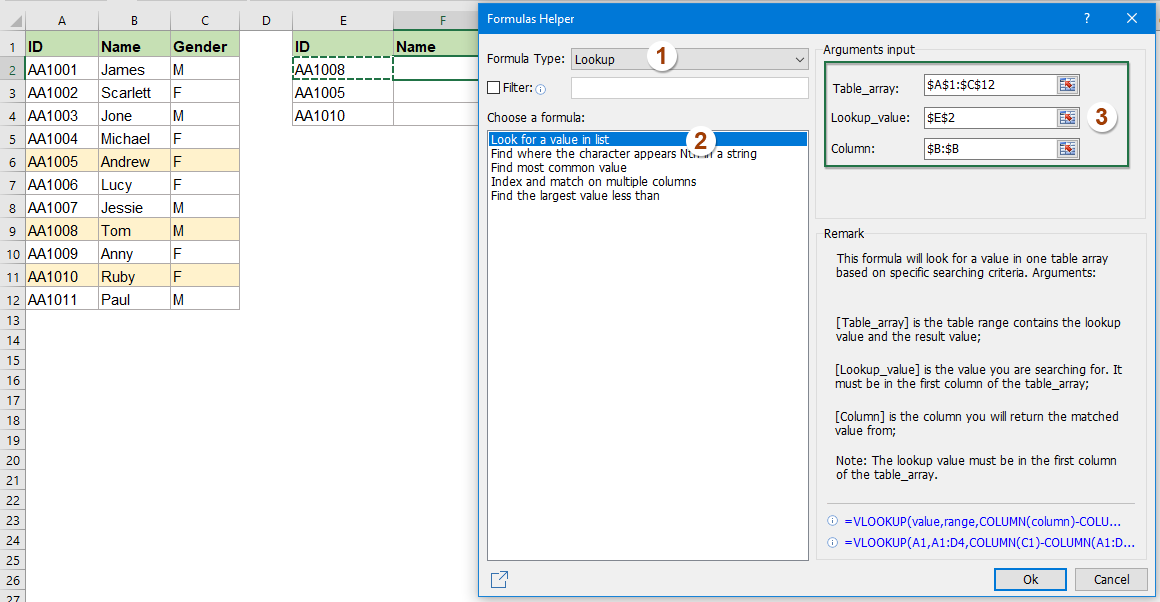4. Then click Ok, and the first matched data based on a specific value has been returned at once. You just need to drag the fill handle to apply this formula to other cells you need,see screenshot: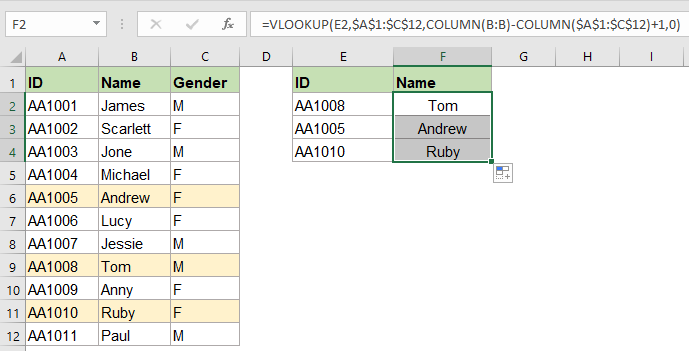Note: If the value is not found in the data range, you will get an error value #N/A.

#### More relative VLOOKUP articles:

• Vlookup And Concatenate Multiple Corresponding Values
• As we all known, the Vlookup function in Excel can help us to lookup a value and return the corresponding data in another column, but in general, it can only get the first relative value if there are multiple matching data. In this article, I will talk about how to vlookup and concatenate multiple corresponding values in only one cell or a vertical list.
• Vlookup And Return The Last Matching Value
• If you have a list of items which are repeated many times, and now, you just want to know the last matching value with your specified data. For example, I have the following data range, there are duplicate product names in column A but different names in column C, and I want to return the last matching item Cheryl of the product Apple.
• Vlookup Values Across Multiple Worksheets
• In excel, we can easily apply the vlookup function to return the matching values in a single table of a worksheet. But, have you ever considered that how to vlookup value across multiple worksheet? Supposing I have the following three worksheets with range of data, and now, I want to get part of the corresponding values based on the criteria from these three worksheets.
• Vlookup Across Multiple Sheets And Sum Results
• Supposing, I have four worksheets which have the same formatting, and now, I want to find the TV set in the Product column of each sheet, and get the total number of order across those sheets as following screenshot shown. How could I solve this problem with an easy and quick method in Excel?

### Best Office Productivity Tools

Easy to Uninstall Completely  |  Supports Office/Excel 2007-2021 and 365  |  Full-Featured 30-Day Free Trial | Available in 44 Languages

 Popular Features: Find/Highlight/Identify Duplicates   |  Delete Blank Rows   |  Combine Columns or Cells without Losing Data   |   Round without Formula ... Super Lookup: Multiple Criteria VLookup  |   Multiple Value VLookup  |   VLookup Across Multiple Sheets   |   Fuzzy Lookup .... Advanced Drop-down List: Quickly Create Drop Down List   |  Dependent Drop Down List   |  Multi-select Drop Down List .... Column Manager: Add a Specific Number of Columns   |   Move Columns   |   Unhide Columns   |   Compare Columns to Select Same & Different Cells ... Featured Features: Grid Focus   |  Design View   |   Big Formula Bar   |  Workbook & Sheet Manager   |  Resource Library (Auto Text)   |  Date Picker   |  Combine Worksheets   |  Encrypt/Decrypt Cells   |  Send Emails by List   |  Super Filter   |   Special Filter (filter bold/italic/strikethrough...) ... Top 15 Toolset:  12 Text Tools (Add Text, Remove Characters, ...)   |   50+ Chart Types (Gantt Chart, ...)   |   40+ Practical Formulas (Calculate age based on birthday, ...)   |   19 Insertion Tools (Insert QR Code, Insert Picture from Path, ...)   |   12 Conversion Tools (Numbers to Words, Currency Conversion, ...)   |   7 Merge & Split Tools (Advanced Combine Rows, Split Cells, ...)   |   Many More...

Kutools for Excel Boasts Over 300 Features, Ensuring That What You Need Is Just A Click Away...

Supercharge Your Spreadsheets: Experience Efficiency Like Never Before with Kutools for Excel  (Full-Featured 30-Day Free Trial)#### Office Tab Brings Tabbed interface to Office, and Make Your Work Much Easier

• Enable tabbed editing and reading in Word, Excel, PowerPoint, Publisher, Access, Visio and Project.
• Open and create multiple documents in new tabs of the same window, rather than in new windows.
• Increases your productivity by 50%, and reduces hundreds of mouse clicks for you every day!